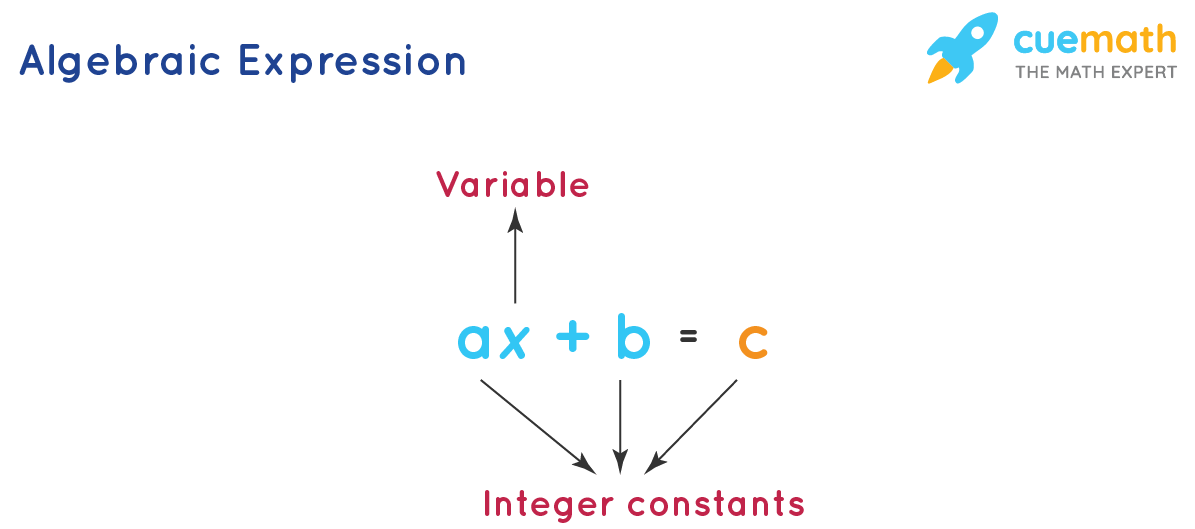# Explain Algebraic Expression With an Example

## Question: Explain Algebraic Expression With an Example

Let us see what an algebraic expression is and how to solve it.

## Answer: An algebraic expression (or) a variable expression is a combination of terms by the operations such as addition, subtraction, multiplication, division, etc.

An algebraic expression consists of variables, constants, and arithmetic operators.## Explanation:

Let us consider my sleep routine for a week:

Monday: I sleep at 9 pm + sleep for 8 hours→ wake up at 5 am

Tuesday: I sleep at 10 pm + sleep for 8 hours→ wake up at 6 am

Wednesday: I sleep at 11 pm + sleep for 8 hours→ wake up at 7 am

So, whatever time I sleep, 8 hours later, I wake up whatever time it is. While the above is a wonderful description using the english language, in the language of mathematics, we have different symbols to denote this relationship.

If we assume the symbol "x" to represent "The time I sleep".

And, let the symbol "y" represent "The time I wake up".

The above relationship between my sleep and wake up time can be translated as

x + 8 = y

The above is a basic algebraic expression consisting of variables (the time I sleep and wake up being variables), constants (8 hours which I sleep), and arithmetic operators like addition, subtraction, multiplication, and division.

Also, in an algebraic expression, terms are the elements separated by the addition or subtraction signs. Terms can consist of variables, coefficients, or constants.

The numbers in the expression or equation are constants. Algebra can include real, complex numbers, matrices, and vectors, etc.

If we transition from Arithmetic to Algebra, it will be like:

Arithmetic: 3 + 4 = 3 + 4, whereas in Algebra it will look like: x + y = y + x